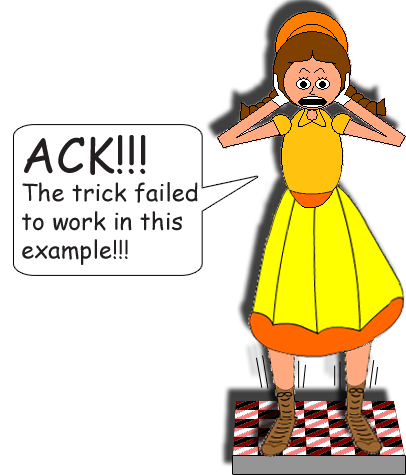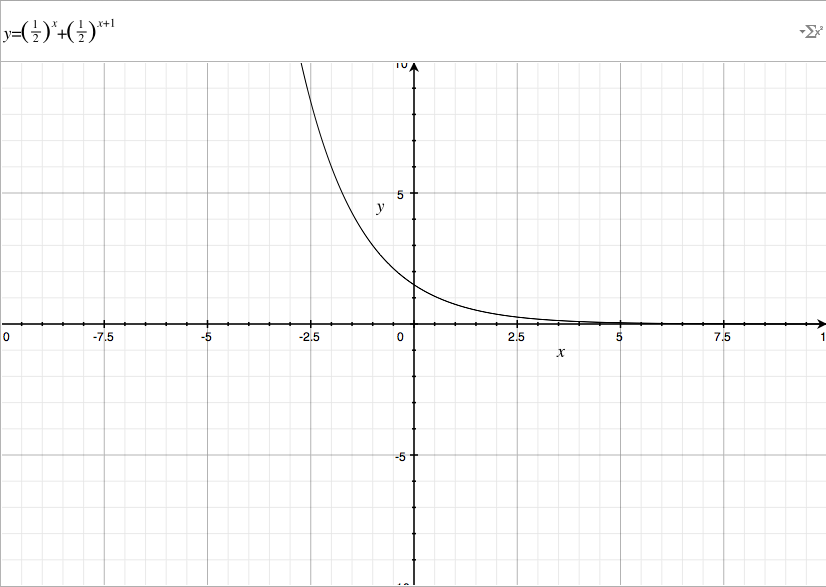# Adding 2 Powers of 1 Half

## 2-x + 2-x-1 = 3 × 2-x-1## Examples:

(½)1 + (½)2 = 1/2 + 1/4 = 3/4

(½)2 + (½)3 = 1/4 + 1/8 = 3/8

(½)3 + (½)4 = 1/8 + 1/16 = 3/16

(½)4 + (½)5 = 1/16 + 1/32 = 3/32

(½)5 + (½)6 = 1/32 + 1/64 = 3/64Even if x = 0, the equation remains true!

(½)0 + (½)1 = 1 + 1/2 = 3/2

You already know that raising any non-zero number to the power of zero gives you 1, right? The equation will still be technically true if x is not an integer, but the sum will not be a rational number! All rational numbers are a quotient of 2 integers!

(½)½ + (½)3/2 = the square root of 1/8 + the square root of 1/2 = 1.060660172...The irrational number in the counterexample is equal to 3 ÷ the square root of 8. Normally in fractions, people write numerators & denominators as integers; although sometimes in advanced algebra & calculus, mathematicians write variables or famous irrational numbers like pi(π) as numerators or denominators! That is done to be more exact! Besides, it'll take forever to write or print all those digits after the decimal point; since there's no digit pattern in irrational numbers, you never know which digit to put next! Most real numbers are irrational!

### Below is how the function looks on a graph:Notice that I printed the function as (½)x + (½)x+1.